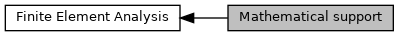Mathematical support

## Description

Collaboration diagram for Mathematical support:## Namespaces

chrono::fea::rotutils
Utility functions for rotations in 3D and their derivatives.

## Classes

class  chrono::fea::ChGaussIntegrationRule
Class for the management of the Gauss Quadrature in 1D, 2D or 3D space. More...

class  chrono::fea::ChGaussPoint
Class for a Gauss point, that has a position (1D-3D) and a weight. More...

class  chrono::fea::ChMatrixCorotation
Perform a corotation (warping) of a K matrix by pre- or post- multiplying it with a C matrix that has 3x3 rotation matrices R as diagonal blocks, so that C*K means: More...

class  chrono::fea::PolarDecomposition
Polar decomposition of a general 3x3 matrix. More...

class  chrono::fea::ChPolarDecomposition< Real >
Perform a polar decomposition of a 3x3 P matrix in order to retrieve the orthogonal Q and the symmetric S form, as P=Q*S. More...

## Functions

template<int N>
bool chrono::fea::LU_factor (ChMatrixNM< double, N, N > &A, ChMatrixNM< int, N, 1 > &INDX, bool &pivoting)
In-place LU factorization. More...

template<int N>
void chrono::fea::LU_solve (const ChMatrixNM< double, N, N > &A, const ChMatrixNM< int, N, 1 > &INDX, ChMatrixNM< double, N, 1 > &B)
LU linear system solution (back substitution) More...

## ◆ LU_factor()

template<int N>
 bool chrono::fea::LU_factor ( ChMatrixNM< double, N, N > & A, ChMatrixNM< int, N, 1 > & INDX, bool & pivoting )

In-place LU factorization.

Return false if the matrix is (close to) singular

Parameters
 [in,out] A matrix to be factorized [out] INDX vector of pivots [out] pivoting true if pivoting was required; false otherwise

## ◆ LU_solve()

template<int N>
 void chrono::fea::LU_solve ( const ChMatrixNM< double, N, N > & A, const ChMatrixNM< int, N, 1 > & INDX, ChMatrixNM< double, N, 1 > & B )

LU linear system solution (back substitution)

Parameters
 [in] A LU factorized matrix [out] INDX vector of pivots [in,out] B on entry, the RHS; on return, the solution vector